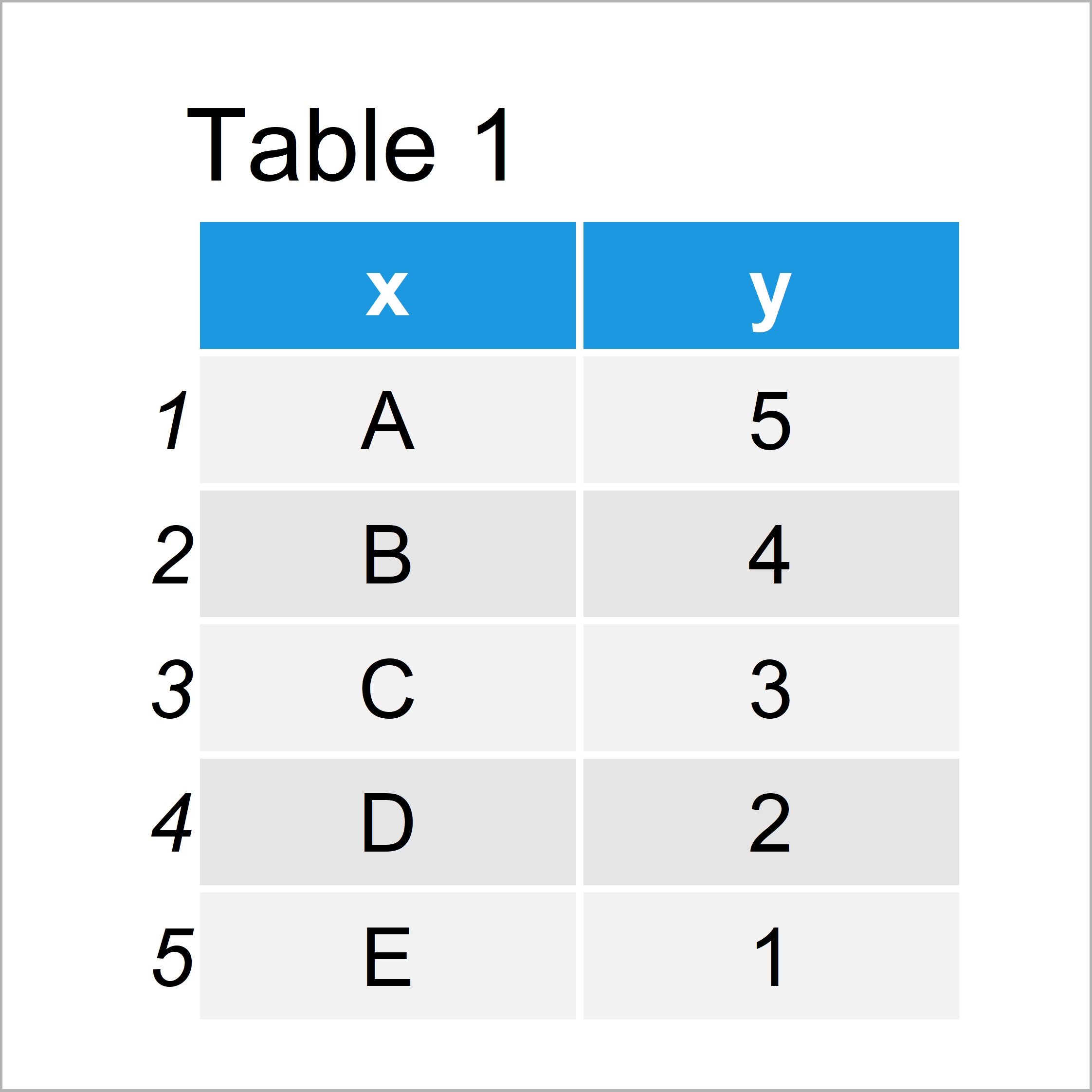# R Error in barplot.default() : ‘height’ must be a vector or a matrix (Example)

In this article you’ll learn how to debug the “Error in barplot.default() : ‘height’ must be a vector or a matrix” in the R programming language.

You’re here for the answer, so let’s get straight to the examples.

## Example 1: Reproduce the Error in barplot.default() : ‘height’ must be a vector or a matrix

This example illustrates how to replicate the error message in the barplot() function: “‘height’ must be a vector or a matrix”.

For this, we first have to create some example data:

```data <- data.frame(x = LETTERS[1:5],    # Create example data
y = 5:1)
data                                    # Print example data```In Table 1 it is shown that we have created a data frame consisting of two columns. The variable x contains the x-axis labels and the variable y contains the height values of our bars.

Let’s try to draw these data with the barplot function:

```barplot(data)                           # Try to create barplot
# Error in barplot.default(data) : 'height' must be a vector or a matrix```

As you can see, the previous R code has returned the “Error in barplot.default(data) : ‘height’ must be a vector or a matrix”.

The reason for this is that we have inserted a data.frame object into the barplot function, i.e. we have specified the height to be equal to our data frame.

However, the height argument of the barplot function only takes numeric vectors or matrices as input.

So how can we solve this problem? That’s what I’ll show next!

## Example 2: Fix the Error in barplot.default() : ‘height’ must be a vector or a matrix

This example shows how to draw a bargraph in R without returning the “Error in barplot.default() : ‘height’ must be a vector or a matrix”.

In order to do that, we have to modify our input data as shown below:

```data_bar <- data\$y                      # Extract values
names(data_bar) <- data\$x               # Assign names to values
data_bar                                # Show new data
# A B C D E
# 5 4 3 2 1```

The previous output of the RStudio console shows how our data looks like. It is a named vector object consisting of the numeric height values and the labels as names of this vector.

Now, we can insert this named vector into the barplot function:

`barplot(data_bar)                       # Draw barplot with names`After executing the previous R programming syntax the barplot shown in Figure 1 has been plotted.

## Video & Further Resources

I have recently published a video on my YouTube channel, which explains the contents of this page. You can find the video below:

In addition, you might have a look at some of the related articles of this homepage:

At this point you should have learned how to handle the “Error in barplot.default() : ‘height’ must be a vector or a matrix” in the R programming language. If you have additional questions or comments, please let me know in the comments below.

Subscribe to the Statistics Globe Newsletter